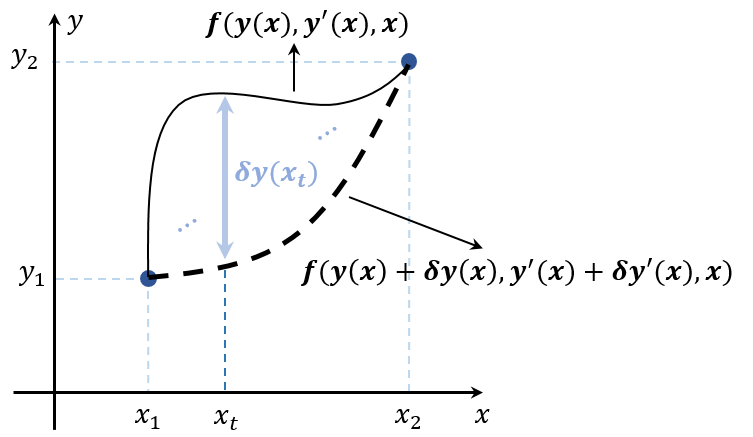# Euler-Lagrange Equation

This equation is useful when finding the critical point of the integral equation. Suppose that $y(x)$ passes through the points $(x_1, y_1)$ and $(x_2, y_2)$ and it is a continuously differentiable function in $[x_1, x_2]$. We want to find $\min \int_{x_1}^{x_2} y(x) dx$ or $\max \int_{x_1}^{x_2} y(x) dx$.

## Step 1. Define the extreme value $F(y)$.

Introducing a path function $f$ which consists of $y(x)$ and $y'(x)$, $F(y)$ can be defined by \begin{aligned} F(y) &= \int_{x_1}^{x_2} f(y(x), y'(x), x) dx \end{aligned}

This function $F$ assumes that $y$ is the extreme value when it follows the path function $f$.Now, consider $F(y + \delta y)$ near the extreme value $F(y)$ with the same start and end points. Then it represents another path function $f$ which consists of $y(x) + \delta y(x)$ and $y'(x) + \delta y'(x)$. By Taylor Theorem, \begin{aligned} F(y + \delta y) &= \int_{x_1}^{x_2} f(y(x) + \delta y(x), y'(x) + \delta y'(x), x) dx \\ &\approx \int_{x_1}^{x_2} f(y(x), y'(x), x) + f_y \delta y + f_{y'} \delta y' + f_x 0 dx \\ &= F(y) + \int_{x_1}^{x_2} f_y \delta y + f_{y'} \delta y'dx \end{aligned}

It yields the following $\delta F$, \begin{aligned} \delta F &= F(y + \delta y) - F(y) \approx \int_{x_1}^{x_2} f_y \delta y + f_{y'} \delta y'dx \end{aligned}

## Step 2. Apply the integration by parts to $\delta F$.

The second part of $\delta F$ can be modified using the integration by parts. \begin{aligned} \int_{x_1}^{x_2} f_{y'} \delta y'dx = [f_{y'} \delta y]_{x_1}^{x_2} - \int_{x_1}^{x_2} \frac{d}{dx}(f_{y'}) \delta y dx = - \int_{x_1}^{x_2} \frac{d}{dx}(f_{y'}) \delta y dx \end{aligned}

This is because that $\delta y(x_1) = \delta y(x_2) = 0$ since $f(y(x), y'(x), x)$ and $f(y(x) + \delta y(x), y'(x) + \delta y'(x), x)$ have the same start and end points. Therefore $\delta F$ is rewritten as \begin{aligned} \delta F \approx \int_{x_1}^{x_2} \left( f_y - \frac{d}{dx} f_{y'} \right) \delta y dx \end{aligned}

## Step 3. Use the fact that $\delta F(y) = 0$.

Since $F(y)$ is the extreme value, $\delta F(y) = 0$ for the small enough $\delta y$. Therefore, it leads to $\begin{gather} \delta F \approx \int_{x_1}^{x_2} \left( f_y - \frac{d}{dx} f_{y'} \right) \delta y dx = 0, \\ \color{red}{f_y - \frac{d}{dx} f_{y'} = 0} \end{gather}$

which is called Euler-Lagrange equation.

### Example: Find the smallest distance between $(x_1, y_1)$ and $(x_2, y_2)$ points.

It is to find the smallest path among all the possible paths. From the step 1, \begin{aligned} \min \int_{x_1}^{x_2} \sqrt{(dx)^2 + (dy)^2} = \min \int_{x_1}^{x_2} \sqrt{1 + y'^2} dx = F(y) \end{aligned}

Now, step 2 and 3 lead to the following Euler-Lagrange equation. \begin{aligned} f_y - \frac{d}{dx} f_{y'} &= 0 - \frac{d}{dx} \left( \frac{y'}{\sqrt{1 + y'^2}} \right) = 0 \\ &\implies \frac{y'}{\sqrt{1 + y'^2}} = C \\ &\implies y' = \pm \sqrt{\frac{C^2}{1 - C^2}} \end{aligned}

where $C \in \mathbb{R}$. It means that $y$ is of form $ax + b$ for $a$, $b \in \mathbb{R}$, $y' = a = \frac{y_2 - y_1}{x_2 - x_2}$. Therefore, \begin{aligned} F(y) &= \min \int_{x_1}^{x_2} \sqrt{1 + y'^2} dx = \int_{x_1}^{x_2} \sqrt{1 + a^2} dx = \sqrt{1 + a^2} (x_2 - x_1) \\ &= \sqrt{1 + \frac{(y_2 - y_1)^2}{(x_2 - x_1)^2}} (x_2 - x_1) = \sqrt{(x_2 - x_1)^2 + (y_2 - y_1)^2} \end{aligned}

This result it right because Euclidean distance is the smallest one between $(x_1, y_1)$ and $(x_2, y_2)$ points.